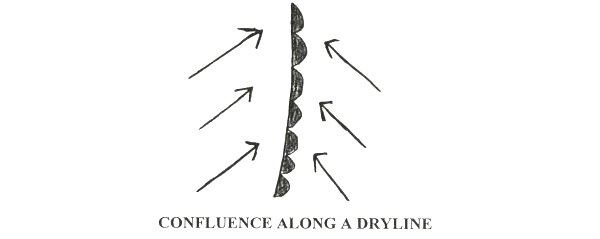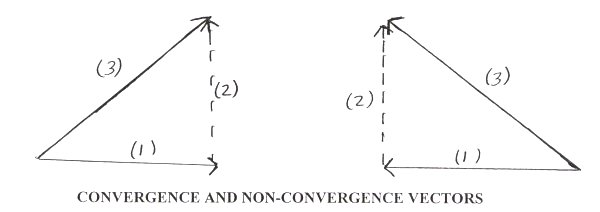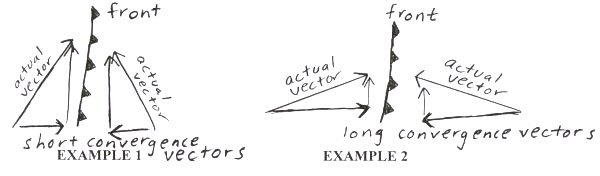[--MAIN HOME--] [--ALL HABYHINTS--] [--FACEBOOK PAGE--]

 MAGNITUDE OF CONVERGENCE AND UVV ALONG A LOW-LEVEL FRONT

METEOROLOGIST JEFF HABY

In order to understand convergence, a basic understanding of vectors is important. A vector displays magnitude and direction. The length of the vector determines it's magnitude, where the vector is pointing represents direction. A longer vector represents a stronger wind speed. Vectors are used on forecast model images. An example of a vector is given below.Convergence occurs as stronger wind flows into weaker wind in the same line of action or results from wind vectors flowing toward each other in the same line of action. An example of each of these is given below.When examining convergence along a front, the wind vectors most likely will not be in the same line of action. Air streams that flow together at an angle to a direct line of action is termed confluence. In the diagram below, the wind is from the southwest to the west of the dryline and from the southeast to the east of the dryline. Confluence is occurring along the dryline. Generally, fronts are zones of confluence (there is a directional change in wind when comparing each side of the front).A confluence vector on each side of a front can be broken down into a convergence and non-convergence component of the vector. The convergence component of the vector is in the same line of action with the convergence vector on the other side of the front while the non-convergence vector is in a non-opposing line of action (90° to convergence vector). A vector on each side of the dryline has been broken down in the diagram below into a convergence and non-convergence vector. (1) is the convergence vector, (2) the non-convergence vector, and (3) the actual wind vector.Only the convergence component of the vectors causes uplift. Low level convergence causes rising air. The non-convergence component of a vector does not cause uplift. Uplift will increase by either (1) increasing the velocity of the convergence vectors or by (2) having the vectors on each side of the front move closer to the same line of action (thus increasing the magnitude of the convergence vector), or (3) a combination of (1) and (2). Examine the two examples below. Each actual wind vector has the same length. Example 2 has better convergence since the convergence components of the vectors are longer.Haby sez: Increasing the amount of convergence along a low-level front increases the synoptic scale UVV along the front.